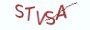Java基础教程

# 6.3.2 break-退出整个循环

break有打破和中途休息的意思，打破循环，意味着终止整个循环。通俗地进行解释，break是循环的终结者，类似T-800机器人一样的存在。

```public class HelloJava{
public  static void main(String[] args) {
int year = 0;
int end = 10000;
while (year < end){
++year;
// 如果变量year的值大于2020就执行break退出循环
if(year > 2020){
break;
}
}
}
}```

```public class HelloJava{
public  static void main(String[] args) {
int end = 10;
int sum = 0;
boolean is_prime_number = true;
for(int number=2; number<=end; ++number){
// 每次迭代时设置is_composite_number为true,即假定当前number为质数
is_prime_number = true;
for(int number_=2; number_ < number; ++number_){
if(number % number_ == 0){
// 只要当前number被整除，那么一定不是质数
is_prime_number = false;
// 执行break只会退出break所在的循环
break;
}
}
// 如果为质数就累加
if(is_prime_number){
sum += number;
}
}
System.out.println("1到10之间的所有质数的和:"+sum);
}```

# 6.3.3 continue-退出本次代码执行

```public class HelloJava{
public  static void main(String[] args) {
int end = 100;
int sum = 0;

for(int number=1; number<=end; ++number){
if(number % 2 == 0)
// 如果number能被2整除，说明是偶数，则执行continue
continue;
/*
(1) 执行continue以后，不会再执行continue下面的代码
(2) 代码能执行到sum+=number,说明number一定是奇数
*/
sum += number;
}
System.out.println("1到100之间的所有奇数的和:"+sum);
}
}```

```public class HelloJava{
public  static void main(String[] args) {
int end = 100;
int sum = 0;

for(int number=1; number<=end; ++number){
if(number % 2 == 1){
// 如果number不能被2整除，说明是奇数，则直接与sum相加
sum += number;
}
}
System.out.println("1到100之间的所有奇数的和:"+sum);
}```

# 6.3.4 return-退出函数执行

6.3.5 课后习题

(1) 谈谈你对转向控制的理解。

(2) Java中通过哪些关键字来编写转向控制？

(3）简述break与continue的主要区别。

(4) 编写一道程序来计算1-100之间的次大质数。

# 6.3.6 高薪就业班

`(1) Python后端工程师高薪就业班，月薪10K-15K，免费领取课程大纲(2) Python爬虫工程师高薪就业班，年薪十五万，免费领取课程大纲(3) Java后端开发工程师高薪就业班，月薪10K-20K, 免费领取课程大纲(4) Python大数据工程师就业班，月薪12K-25K,免费领取课程大纲`## 注册账号